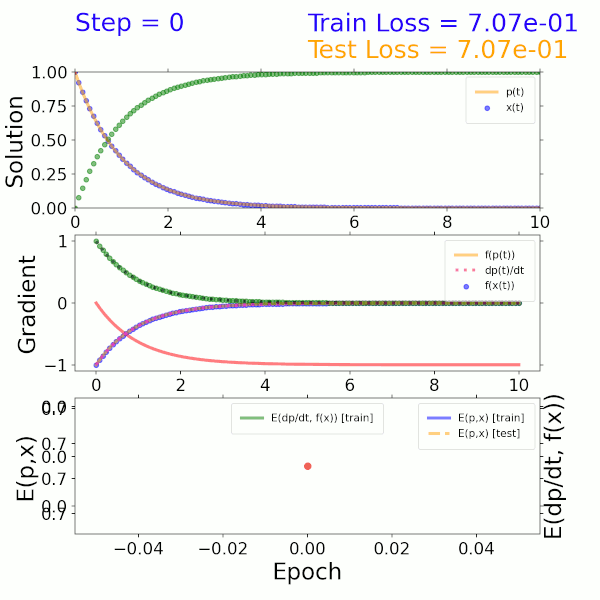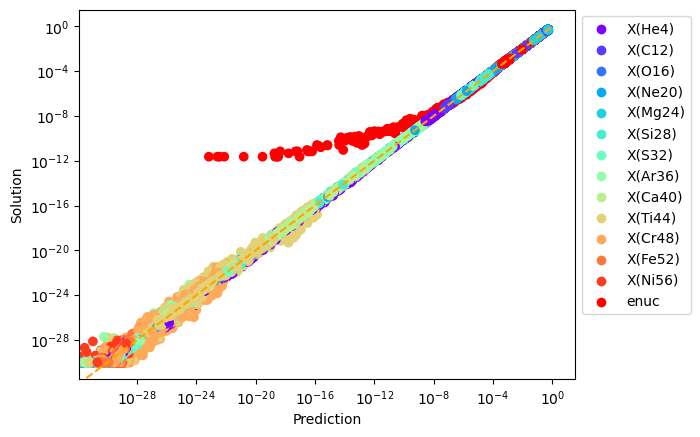# Machine Learning Accelerated Models

## CCSE Members of the Machine Learning Development Team

• Doreen Fan

• Andy Nonaka

• Hengjie Wang

• Donald Willcox

• The goal of using machine learning models within an AMReX framework is to accelerate the time-to-solution of computational kernels without reducing accuracy.

# Using pyTorch Models in AMReX

We have developed an interface to load a pyTorch-trained machine learning model into an AMReX simulation to replace a computational kernel. A guided tutorial including a two-component beta decay system is available HERE.As a proof of concept, a surrogate model was trained using the solution to a two-component ODE system describing beta decay. The input is a time step and output is the solution representing the mass fractions of the two species. The model itself consists of a dense neural network (DNN) that imposes both mass fractions and mass fraction rates (gradients) as constraints in the loss function. As shown in the solution (top), gradient (middle), and error (bottom) plots on the left, this model converges to the exact solution after approximately 300 epochs.

# Surrogate Model Development for StarKiller Reaction Networks

Our goal is to use a machine learning model to replace the reaction network in an astrophysical simulation. We perform smallscale flame simulations with MAESTROeX using the aprox13 network in the StarKiller Microphysics, which is used in astrophysical simulations including stellar explosions (please refer to Astrophysics and Cosmology for more details) Traditionally, we have used stiff ODE integrator for reactions, in which case the reactions dominate the total runtime. Preliminary tests have shown that the use of a ML model can reduce the runtime by an order of magnitude. Below is a normalized plot of the prediction vs. solution for a number of species and the nuclear energy generation rate. The model was trained by sampling a large fraction of cells in a traditional simulation, and generating the error plot with the unused training data.Current development of machine learning capabilities for reaction networks can be found on our GitHub repo at https://github.com/AMReX-Astro/ml-reactions.